# Uniform Circular Motion and Centripetal Acceleration (non-intuitive)

• babaliaris
In summary, acceleration is the rate of change of velocity, and in uniform circular motion, it describes how fast the direction of velocity changes. This is different from projectile motion, where acceleration describes how fast the magnitude of velocity changes. When taking a turn in a car, the feeling of being pushed in the opposite direction of the circle's center is actually due to a force from the car's seat pushing you towards the centripetal acceleration, and your body pushing back in the opposite direction due to Newton's 3rd law.f

#### babaliaris

I'm in the chapter of Uniform Circular Motion and I have a hard time understating centipetal acceleration. Until now I knew that acceleration describes "how fast velocity changes in magnitude" except projectile motion because when it reaches maximum height then g is going to change the direction on the Vy and make it move downwards but now in this kind of motion, acceleration describes only "how fast the direction of the velocity changes" and not the magnitude. Ok I understand that but what I really don't understand is when I'm in a car and take a turn I feel pushed in the opposite direction of the circles center. But acceleration is pointing at the center of the circle

Any change of the velocity (a vector!) means that there's non-zero acceleration, because by definition
$$\vec{a}(t)=\dot{\vec{v}}(t)=\ddot{\vec{x}}(t).$$
For the uniform motion on a circle (for convenience I put it in the ##xy##=plane of the coordinate system) you have
$$\vec{x}(t)=r [\vec{e}_x \cos (\omega t) + \vec{e}_y \sin(\omega t)].$$
Now you can easily evaluate velocity and acceleration.

Then, using Newton's 2nd law you immediately get which force you have to apply to the particle to make it move in the described way along a circle.

Last edited:
•babaliaris
Until now I knew that acceleration describes "how fast velocity changes in magnitude"
No - acceleration is how fast velocity vector is changing (or, more precisely, the rate of change of the velocity vector). The magnitude only changes sometimes; the direction only changes sometimes. The vector always changes. I remember feeling weird about that too, but it must be that way. There's a force acting on a mass (e.g. a string pulling on a stone being swung around in a circle), and ##F=ma## tells you there must be an acceleration.
Ok I understand that but what I really don't understand is when I'm in a car and take a turn I feel pushed in the opposite direction of the circles center.
There are two ways of looking at this, and you are mixing them up.

The first way to look at a car going round a corner is from outside it. I'm standing on a bridge above the road, looking down on your car as you start to corner. What I see is that the car is cornering, but your body is trying to carry on in a straight line (Newton's First Law). But if you carry on in a straight line, the car door curves into you and presses you into a curve. That's the centripetal force, and it's my explanation for why you turn.

The second way to look at it is from inside the car. From this perspective, the world is turning and the car isn't moving. But you still need an explanation of why the door is pressing into you - mine doesn't make sense because the door doesn't turn in this perspective. Your explanation is the centrifugal force (sometimes called a fictitious force, or an inertial force) that just comes out of nowhere and presses you to the door.

The book is using the first perspective. It's mathematically a lot simpler to handle, but it's a bit counter-intuitive since we're seldom doing detailed analysis of the kinematics of a car passing underneath a bridge. The second explanation is the natural one for us since we're used to traveling in cars, but it involves more complex maths to explain what inertial forces are so physics textbooks tend to avoid it until further on in your education. I think I was taught it in my second year at university (although I don't think I grasped the significance until much later...)

The problem is that many books do not use the really simple mass, as I suggested to use in #2. Some textbook make very simple things very complicated by a misguided attempt to "avoid math". It should be very clear that math is helping to make things much simpler and cannot be avoided anyway. So one should introduce the appropriate math right away when it's useful for a given problem, rather than avoiding it. For mechanics you need vector algebra, derivatives and integrals as well as ordinary differential equations.

•babaliaris
That's true , you feel LIKE there is a force that is pushing you to the opposite direction of the centripetal acceleration.

But what you actually feel, is a force from the car's seat mainly, that is pushing you towards centripetal acceleration (so the force on your body from the car's seat is on the same direction as centripetal acceleration), and due to Newton's 3rd Law, your body is pushing the car's seat with an opposite force, that is your body is pushing the car's seat in a direction opposite to centripetal acceleration. That push from your body to the car's seat is what your wrongly understand as "being pushed in direction opposite of the centripetal acceleration".

•babaliaris and vanhees71
I'm in a car and take a turn I feel pushed in the opposite direction of the circles center. But acceleration is pointing at the center of the circle
When the car accelerates forward, you end up in the back of the car, if you are not fixed an thus fail to accelerate with the car. The same applies to accelerating left or right in a turn.

•babaliaris and vanhees71
I'm a little confused... Well I still haven't reached the chapter about forces and Newton's laws. Now I'm in the relative motion chapter and next is the forces and motion chapter and Newton's first law. Do you thing I will have a better understating after that chapter?

When the car accelerates forward, you end up in the back of the car, if you are not fixed an thus fail to accelerate with the car. The same applies to accelerating left or right in a turn.
Best reply! Now I see! Well I can't wait to reach the chapter of forces and Newton's laws to get a deeper understanding!

I'm a little confused... Well I still haven't reached the chapter about forces and Newton's laws. Now I'm in the relative motion chapter and next is the forces and motion chapter and Newton's first law. Do you thing I will have a better understating after that chapter?
Yes, in my opinion you absolutely need a good understanding of Newton's 2nd and 3rd law to fully understand what's going on.

•Ibix
Yes, in my opinion you absolutely need a good understanding of Newton's 2nd and 3rd law to fully understand what's going on.

•Delta2
That's true , you feel LIKE there is a force that is pushing you to the opposite direction of the centripetal acceleration.

But what you actually feel, is a force from the car's seat mainly, that is pushing you towards centripetal acceleration (so the force on your body from the car's seat is on the same direction as centripetal acceleration), and due to Newton's 3rd Law, your body is pushing the car's seat with an opposite force, that is your body is pushing the car's seat in a direction opposite to centripetal acceleration. That push from your body to the car's seat is what your wrongly understand as "being pushed in direction opposite of the centripetal acceleration".

Your answer make's the most sense now (i finished reading about uniform circular motion), but still i can't understand a few things. It's weird, i reached so far learned about motions, forces, Newton's laws and now I've stuck... When a particle move's in a uniform circular motion there is a Centripetal force towards the center.

Question 1:
So if we assume there is no gravity and no other forces applied on the particle, why the particle is not moving towards the center of the circle? So if I name r the axis where the Centripetal force is lying , according to Newton's second law:

∑ƒr = m⋅a ⇔ -ƒc = m ⋅ ( -v^2 / R ) ⇔ fc = m ⋅ ( v^2 / R ) but still i can't understand why the parcicle is moving in a circle and not towords the center. If you can use maths to explain use them.

Question 2:
Let's say i have a rope tied in a particle and I'm spinning it (around a y axis) with my hand. Then the particle is entering a uniform circular motion but also it does not fall to the ground and according to Newton there must be another force with the same magnitude as Fg (weight) in the opposite direction so the particle won't move at all on the y axis. Also if i speed faster and increase the constant speed of the uniform circular motion, the rope will break. Consider the following sketch: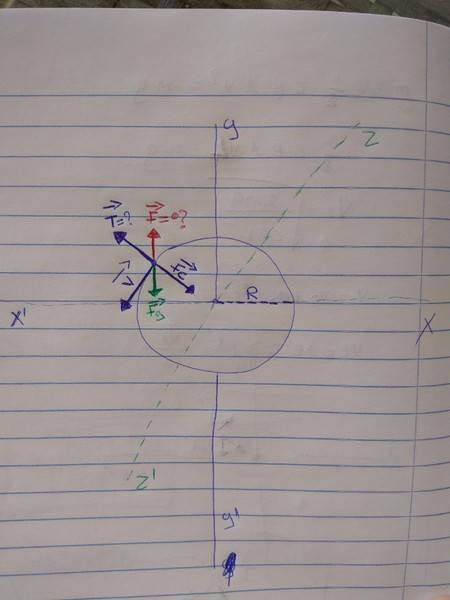I have a really hard time understanding the cause of these forces. It must F = -Fg so the particle won't fall to the ground or actually won't move to the y-axis at all! There must be also a T force that when it gets high enough it will break the rope. Can someone explain them to me? In this scenario does the T force of the rope being related to the centripetal force?

#### Attachments

What's wrong with my suggestion in #2? It's really not more to the kinematics of circular motion than this very simple mathematical exercise!

•babaliaris
What's wrong with my suggestion in #2? It's really not more to the kinematics of circular motion than this very simple mathematical exercise!
x(t)=r[excos(ωt)+eysin(ωt)]

I can't understand how to relate the formula above with the forces. Also its a little hard for me to understand what the formula actually describes. Probably an orbit around the circle (position)? Maybe because I don't think "multidimensionaly"? I see a force (pulling the particle towards the center of the circle) and I'm wondering why it does not moving towards the center? Is it because I'm thinking that the force is being applied in on dimension? Please also consider the sketch i uploaded in #11

Last edited:
Ok, so maybe I did not understand at which level you are studying. You marked the thread as to be I level. So I assumed you know vectors and calculus.

##\vec{x}(t)## describes the trajectory of the particle in 3D space, i.e., it gives the position in terms of a vector pointing from some fixed origin of your reference frame as a function of time. If you take a Cartesian basis system ##\vec{e}_j## (##j \in \{1,2,3 \}##), i.e., three unit vectors that are mutually perpendicular to each other, you can describe the vector by three real numbers, its components with respect to this Cartesian basis, which are functions of time:
$$\vec{x}(t)=\sum_{j=1}^3 x_j(t) \vec{e}_j.$$
Now the motion along a circle with constant angular velocity ##\omega## is described by the above given formula
$$\vec{x}(t)=r [\vec{e}_1 \cos(\omega t) + \vec{e}_2 \sin(\omega t)], \quad r>0 \quad \text{constant}.$$
Now the 1st derivative with respect to time gives the velocity,
$$\dot{\vec{x}}(t)=\vec{v}(t)=r \omega [-\vec{e}_1 \sin(\omega t) + \vec{e}_2 \cos(\omega t)].$$
The second derivative is the acceleration
$$\ddot{\vec{x}}(t)=\dot{\vec{v}}(t)=\vec{a}(t)=-r \omega^2 [\vec{e}_1 \cos(\omega t) + \vec{e}_2 \sin(\omega t)]=-\omega^2 \vec{x}(t).$$
Now according to the 2nd law, this implies that you need a force
$$\vec{F}=m \vec{a}=-m \omega^2 \vec{x}.$$
This tells you that the force has magnitude $$F=|\vec{F}|=m \omega^2 r=\text{const}$$, and at any instant of time points radially inwards, i.e., the force pulls the mass instantly towards the origin of the coordinate system, around which it rotates, to keep it on its circular orbit.

•Delta2 and babaliaris
Ok, so maybe I did not understand at which level you are studying. You marked the thread as to be I level. So I assumed you know vectors and calculus.

##\vec{x}(t)## describes the trajectory of the particle in 3D space, i.e., it gives the position in terms of a vector pointing from some fixed origin of your reference frame as a function of time. If you take a Cartesian basis system ##\vec{e}_j## (##j \in \{1,2,3 \}##), i.e., three unit vectors that are mutually perpendicular to each other, you can describe the vector by three real numbers, its components with respect to this Cartesian basis, which are functions of time:
$$\vec{x}(t)=\sum_{j=1}^3 x_j(t) \vec{e}_j.$$
Now the motion along a circle with constant angular velocity ##\omega## is described by the above given formula
$$\vec{x}(t)=r [\vec{e}_1 \cos(\omega t) + \vec{e}_2 \sin(\omega t)], \quad r>0 \quad \text{constant}.$$
Now the 1st derivative with respect to time gives the velocity,
$$\dot{\vec{x}}(t)=\vec{v}(t)=r \omega [-\vec{e}_1 \sin(\omega t) + \vec{e}_2 \cos(\omega t)].$$
The second derivative is the acceleration
$$\ddot{\vec{x}}(t)=\dot{\vec{v}}(t)=\vec{a}(t)=-r \omega^2 [\vec{e}_1 \cos(\omega t) + \vec{e}_2 \sin(\omega t)]=-\omega^2 \vec{x}(t).$$
Now according to the 2nd law, this implies that you need a force
$$\vec{F}=m \vec{a}=-m \omega^2 \vec{x}.$$
This tells you that the force has magnitude $$F=|\vec{F}|=m \omega^2 r=\text{const}$$, and at any instant of time points radially inwards, i.e., the force pulls the mass instantly towards the origin of the coordinate system, around which it rotates, to keep it on its circular orbit.

LOL. I'm a university student and I know calculus and vectors. The problem was in the symbols (I'm such an indiot :p ). Because in greece we use i,j and k as the normalize vectors of the Cartesian system I did not realize that e1 and e2 was actually the normalize vectors (did't even noticed the arrows above them)and thus i did not understand that the formula was actually producing a vector... And i was saying to myself "how can a scalar size give the position of an orbit?" Now i understand! I really appreciate your help! I can't understand why the book is hiding this formula, everything seems much easier now.

So, can you know explain why when you are spinning a particle that is tied in a rope (so the particle is doing a uniform circular motion) why it does not fall to the ground due to it's weight? Also if the speed of the uniform circular motion is big enough it can break the rope, why is that? Also i want to ask something else. In the book: a = v^2 / R (this is the magnitude only) .The ω which u use in the formula above is something else than V? Why the book does not mention something about ω when it should?

So, can you know explain why when you are spinning a particle that is tied in a rope (so the particle is doing a uniform circular motion) why it does not fall to the ground due to its weight?
Consider a projectile. The only force on it, the force of gravity, points down, but it doesn't fall straight down as you seem to suggest the spinning particle should. Why is that?

Consider a projectile. The only force on it, the force of gravity, points down, but it doesn't fall straight down as you seem to suggest the spinning particle should. Why is that?
I don't knowThe projectile moves horizontally with a constant velocity but vertically accelerates with a = -g, so it will hit the ground sometime. The spinning particle accelerates all the time and moves in an orbit where it's velocity changes due to the acceleration. Maybe this is the reason? But there is no way to see it with mathematics? I can't just believe words... There's must be a force on the particle except it's weight (Fg) so ∑ƒy = 0 ⇔ -m⋅g + F = 0 ⇔ F = m⋅g and maybe this F has to due something with the Centripetal force. Probably I'm wrong but this the most logical thing to think of.

Or better, take this example: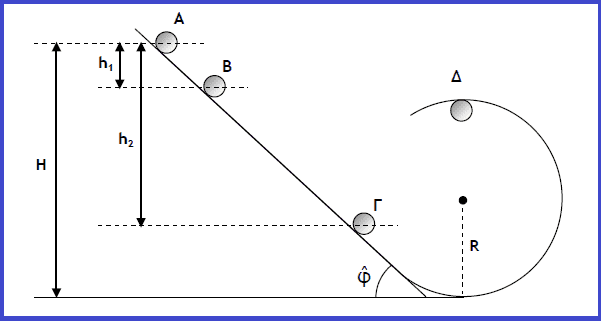When the ball is upside down then the forces which are being applied to it are it's weigh, the normal force and the centripetal force so : -ƒc - m⋅g - ƒn = m⋅ay, in other words if the ball does not fall it means ay ≠ 0 and actually ay > 0. According to Newton if there is an ay > 0 (towards the sky) then it must be also a force in the same direction in such a way -ƒc - m⋅g - ƒn + F = 0 <=> F = ƒc + m⋅g + ƒn

Honestly I can't think of something else...

#### Attachments

Last edited:
Your answer make's the most sense now (i finished reading about uniform circular motion), but still i can't understand a few things. It's weird, i reached so far learned about motions, forces, Newton's laws and now I've stuck... When a particle move's in a uniform circular motion there is a Centripetal force towards the center.

Here is how I like to explain it...

Consider a particle moving in a straight line. To deflect it off course slightly you need to give it a brief sideways force/nudge. Afterwards its still moving in a straight line but in a different direction. Give it another nudge and it changes direction again. With care you could make it move in a square pattern, pentagon, hexagon or octagon. The more sides the shape has the more nudges you need to give it and the more frequently you need to give them. In the extreme (eg a circle) you need to give it a constant sideways force. This is called the centripetal force.

You can calculate the centripetal force _required_ to move in a circle of radius r at velocity v...

F = mv2/r

Question 1:
So if we assume there is no gravity and no other forces applied on the particle, why the particle is not moving towards the center of the circle?

If you apply a force greater than mv2/r to the particle it WILL move toward the centre. You can rearrange the equation to..

r = mv2/F

If F increases the radius r reduces. If F reduces the radius r increases.

Note that F is the net force on the particle. In some cases the net force has two components, gravity might be one and string tension or normal force from a track the other.

•babaliaris and Delta2
So, can you know explain why when you are spinning a particle that is tied in a rope (so the particle is doing a uniform circular motion) why it does not fall to the ground due to it's weight? Also if the speed of the uniform circular motion is big enough it can break the rope, why is that? Also i want to ask something else. In the book: a = v^2 / R (this is the magnitude only) .The ω which u use in the formula above is something else than V? Why the book does not mention something about ω when it should?

You mean spin it in a vertical circle?

At all times the net force must be mv2/r otherwise it won't move in a circle.

At the top "Falling down" requires the radius to reduce. In order for that to happen the centripetal force must be greater than mv2/r.

That will happen if gravity provides too much centripetal force. Another way to say the same thing is... Gravity will provide too much centripetal force (and it will fall) if the velocity is too slow.

•babaliaris
In the book: a = v^2 / R (this is the magnitude only) .The ω which u use in the formula above is something else than V?
ω is angular velocity. It's often used in problems dealing with circular motion. Just as regular velocity is the change in distance over time, ω is the change in angle over time.
The relationship between the two is ##V = ωR##.
Best to visualise by considering a particle going around in a circle of radius R, with the period of one full rotation being T. Then the magnitude of its velocity is the distance covered in one rotation (##d=2πR##) over the period T. I.e., ##V=\frac{2πR}{T}##.
At the same time, you can say that its angular velocity is the angle covered in one rotation (ε = 360 degrees, or 2π in radians) over one period T. I.e. ##ω = \frac{2π}{T}##
Divide V by ω to get to the ##V = ωR##. Which is intuitively right, since if you want to cover the same angle per unit time in a larger circle, you need to go at higher speed.

Also if the speed of the uniform circular motion is big enough it can break the rope, why is that?

To go fast requires a large centripetal force because the required centripetal force is proportional to v squared. Most of it is provided by tension in the string. At the bottom string tension has to counter gravity AND provide the centripetal force. At the top gravity helps provide some centripetal force so it's more likely to fail at the bottom.

Your answer make's the most sense now (i finished reading about uniform circular motion), but still i can't understand a few things. It's weird, i reached so far learned about motions, forces, Newton's laws and now I've stuck... When a particle move's in a uniform circular motion there is a Centripetal force towards the center.

Question 1:
So if we assume there is no gravity and no other forces applied on the particle, why the particle is not moving towards the center of the circle? So if I name r the axis where the Centripetal force is lying , according to Newton's second law:

∑ƒr = m⋅a ⇔ -ƒc = m ⋅ ( -v^2 / R ) ⇔ fc = m ⋅ ( v^2 / R ) but still i can't understand why the parcicle is moving in a circle and not towords the center. If you can use maths to explain use them.

Question 2:
Let's say i have a rope tied in a particle and I'm spinning it (around a y axis) with my hand. Then the particle is entering a uniform circular motion but also it does not fall to the ground and according to Newton there must be another force with the same magnitude as Fg (weight) in the opposite direction so the particle won't move at all on the y axis. Also if i speed faster and increase the constant speed of the uniform circular motion, the rope will break. Consider the following sketch:

I have a really hard time understanding the cause of these forces. It must F = -Fg so the particle won't fall to the ground or actually won't move to the y-axis at all! There must be also a T force that when it gets high enough it will break the rope. Can someone explain them to me? In this scenario does the T force of the rope being related to the centripetal force?
A particle's acceleration ##\vec{a}## can be split to two components ##\vec{a}(t)=\vec{a}_{tan}(t)+\vec{a}_c(t)##.

1)##\vec{a}_{tan}(t)## is the component of acceleration that is always (at any instant of time) parallel to the vector of velocity, and what it does is to change the magnitude of the vector of velocity. It is called tangential acceleration. This component cannot change the direction of the velocity vector, simply because it is always, that is at any instant of time parallel to the velocity vector and we know when an acceleration is parallel to velocity, all it does is to change the magnitude of velocity.

2)##\vec{a_c}(t)## is the component of acceleration that is always perpendicular to the vector of velocity, and what it does is to change the direction of the vector of velocity. It is called centripetal acceleration. This component cannot change the magnitude of the velocity vector simply because it is always, that is at any instant of time perpendicular to the vector of velocity.

I understand that the way I split the total acceleration and the comments of 1) and 2) are not fully mathematically rigorous (for example you might ask, why the centripetal acceleration changes the direction of the velocity vector and only that). If you want the full mathematical reasoning, I ll provide it, just ask.

In uniform circular motion, the magnitude of tangential acceleration is constant and zero. The magnitude of centripetal acceleration is as you know , constant ##\frac{v^2}{R}## and post #14 very successfully illustrated all the math behind its calculation.

The equation that must hold between centripetal and tangential acceleration so that we have circular (uniform or non uniform motion) is

##|\vec{a}_c(t)|=\frac{({\int |\vec{a}_{tan}(t)|dt})^2}{R} (1)##

for some constant R (I can provide the math of how we arrive at this equation, it is a generalization of what vanhees71 does at post#14).

So :
Question 1: The only acceleration is centripetal acceleration, that is always (that is at any instant of time), vertical to the vector of velocity, so all that can do is just change the direction of the velocity vector and not its magnitude. Notice that centripetal acceleration here, is not the same as the vertical acceleration in a particle that does projectile motion. In the projectile case the gravitational acceleration is vertical to velocity only at the start, so it acts as centripetal acceleration only at start, but afterwards the gravitational acceleration it can be split to two components, one tangential and one centripetal. The reason that it doesn't do circular motion is that if you do analyse the force and the acceleration into tangential and centripetal component you ll find that equation (1) cannot be satisfied for some constant R, R has to be time varying.

Question 2: In this case, both the centripetal acceleration and the tangential acceleration are time varying. The particle does non uniform circular motion.
if we do analyze the forces correctly into parallel and perpendicular to the velocity, components, we ll find that the tangential acceleration is ##a_{tan}(t)=g\cos{\theta(t)}##. The centripetal acceleration is ##a_c(t)=\frac{T(t)}{m}+g\sin{\theta(t)}## where T(t) the tension of the string which will be time varying. Why equation (1) is true in this case? The key is the tension of the string, which can change and adjust itself so that equation (1) is satisfied. Of course if that integral in (1) (which is essentially the time varying magnitude of velocity)becomes two big then the string might break because it will not be able to supply such a big T(t).. or if it becomes two small, then T(t) might have to become radially outwards (we know that a string can supply only a radially inwards force).

Last edited:
•babaliaris
i can't understand why the parcicle is moving in a circle and not towords the center.
Do you understand the difference between velocity and acceleration? Accelerating towards the center is different from moving towards the center.

Can someone explain them to me? In this scenario does the T force of the rope being related to the centripetal force?
If it moves in a circle the net force is the centripetal force mv2/r, and the string tension will be whatever is needed for that.

•babaliaris
I think you need to study vector algebra and analysis once again. This is really very basic stuff, and without this mathematical prerequisites there's no way to learn physics on the university level (in my opinion not at any adequate level)!

•babaliaris
Guys thank you very much! I understood everything! The problem was not in lack of mathematical concepts but because I was probably overthinking and overcomplicating things too much. I was thinking that the direction of forces define the direction of the motion something that of course is wrong. Then i started thinking one by one what is a force, what is acceleration and what is velocity and by combining them with your answers I finally got over the overthinking. So this is my conclusion:

1) A force is just a property which when it exist's in an object with mass, then this object accelerates.
2) Velocity is the change of distance over time.
3) Acceleration is the change of Velocity (change in direction or magnitude or both).

Key Problem: FORCES DO NOT SHOW THE DIRECTION OF THE MOTION (I was overthinking to much obviously, reading a lot of hours too because I'm hungry to learn!)

So a particle that is moving straight with a constant speed does not change directions. In a uniform circular motion, the particle must accelerate all the time so velocity will always change in direction but not speed. The particle does not fall on the ground, because if it was then it means that is not in a uniform circular motion or in other words the velocity has changed in direction in such a way that now the direction of the Velocity is always pointing downwards, but this can only happen when there is no centripetal acceleration so the direction of the particle's velocity won't change over time but remains towards the ground due to a = -g acceleration.

•vanhees71 and Delta2
Now I have one more question. Consider the following: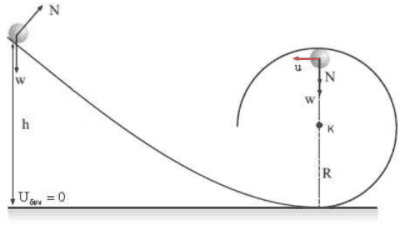The ball is upside down. The information which i have is:
1) The speed of the ball (lets say that the speed is constant when it starts the circular motion).
2) The mass of the ball.
3) The radius of the circle.

Find the normal force when the ball is upside down.

According to the book, the only things that i can calculate so far (what i learned in physics so far) is ONLY the magnitude of the centripetal force and the weight of the ball. Because in this situation all the forces are parallel to the y-axis and point downwards i can write:
-m⋅g - Fn = m⋅(-u^2 / R)

I know everything except Fn so i can calculate it. Now the book does not showing other situations, maybe because i don't have the knowledge yet, but what if I was analyzing the same problem when the ball was a little more to the right than it is now.

In this situation i know that weight = -m⋅g but I don't know the direction of the acceleration. I can calculate the magnitude but how am i going to find the direction? If i just knew the angle of the centripetal acceleration with the x-axis i could calculate the vector but now can i do something? Or the exercise should give me one more information, perhaps the angle of a with x at that point.

#### Attachments

The diagram looks like the initial height, h, is equal to 2R. Is that true? If you know you can figure out the velocity, v, by conservation of the initial energy. Otherwise, you would need to express v as a function of the variable h, v(h).

I don't understand your question about the acceleration of gravity. It is down. When the ball is at the top, the centrifugal acceleration is up and the acceleration of gravity is directly opposite.

Last edited:
In this question, there's no reason to got into non-inertial frames. Just stay in the "lab frame", and there's no centrifugal force (but a centripetal force!).

Sorry guys my mistake, i gave you wrong image.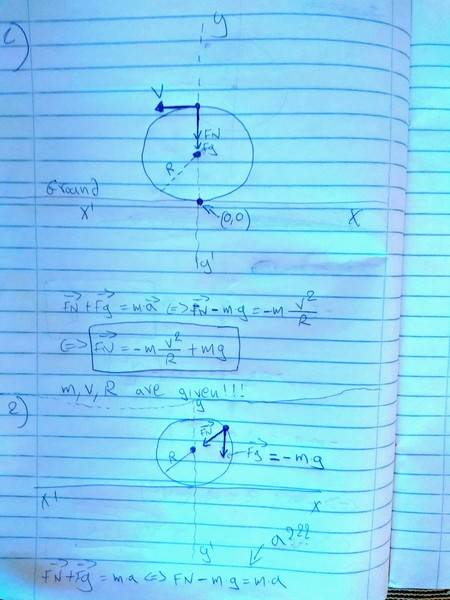In the second (i forgot to draw the Velocity vector but the particle is doing uniform circular motion inside a ring), a should have the same direction as Fn (towards the center) but i can only calculate the magnitude of a = v^2 / R and thus i can't find Fn because i don't have enough info about the a vector. (Also in Newtons second law i forgot to put an arrow above a at the bottom of the page and Fn, a and Fn are vectors there)

#### Attachments

Last edited:
The vector of centripetal acceleration at a point has always the direction of the radius at that point. So in your scheme, the vector of centripetal acceleration points towards the center, has the same direction as ##\vec{F_N}##.

•babaliaris
The vector of centripetal acceleration at a point has always the direction of the radius at that point. So in your scheme, the vector of centripetal acceleration points towards the center, has the same direction as ##\vec{F_N}##.

Yes but i don't know Fn I want to calculate it. Please also consider #30 (because i provided you with the wrong image on #27)

yes I war referring to the second scheme in post #30. The centripetal acceleration there has the same direction as ##\vec{F_N}##. But the total acceleration which is ##\vec{a}=\vec{a}_{tangential}+\vec{a}_{centripetal}## makes an angle with the x-axis which you can calculate, together with the calculation of ##F_N##

•babaliaris
yes I war referring to the second scheme in post #30. The centripetal acceleration there has the same direction as ##\vec{F_N}##. But the total acceleration which is ##\vec{a}=\vec{a}_{tangential}+\vec{a}_{centripetal}## makes an angle with the x-axis which you can calculate, together with the calculation of ##F_N##
I can't understand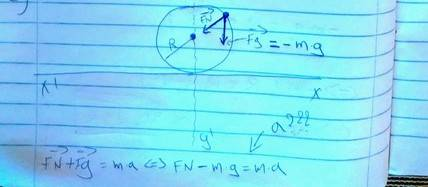This is where i reached so far: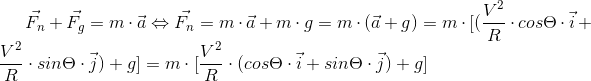I know v, m, r and g but not theta. I need theta to calculate the final result...

[Edited]
LoL actually i can find the magnitude of Fn when the particle is upside down and then in the formula above a can replace Fn = |Fn| (cos8 i + sin8 j) and now i have to find (cos8 i + sin8 j) . This is what you meant(8 = theta symbol)

#### Attachments

Last edited:
First of all ##\Theta## can be calculated if you know the height h of that point, it will be ##\sin\Theta=\frac{h}{R}##

Your equation is correct at start but at some point it goes wrong. The total acceleration is not only the centripetal acceleration. There is tangential acceleration too. You correctly expanded centripetal acceleration to its i, j components, but you need to add the tangential acceleration components too. So you missing two terms. You also forgot to write g as ##g\vec{j}##.